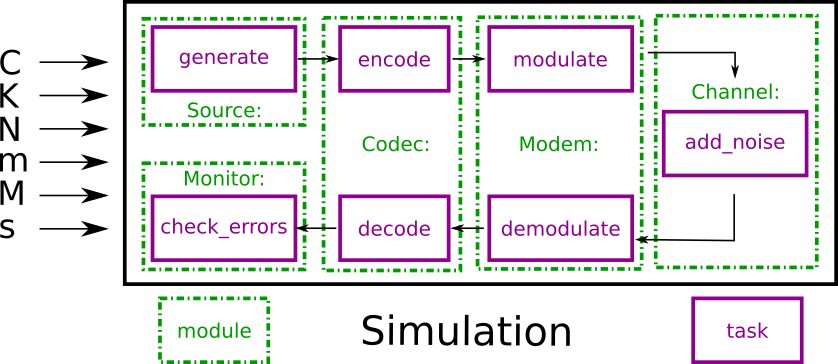# 3.1. Overview¶

The AFF3CT toolbox comes with a simulator dedicated to the communication chains. The simulator focuses on the channel coding level. It can be used to reproduce/validate state-of-the-art BER/FER performances as well as an exploration tool to bench various configurations.

The AFF3CT simulator is based on a Monte Carlo method: the transmitter emits frames that are randomly noised by the channel and then the receiver try to decode the noised frames. The transmitter continues to emit frames until a fixed number of frame errors in achieved (typically 100 frame errors). A frame error occurs when the original frame from the transmitter differs from the the receiver decoded frame. As a consequence, when the SNR decreases, the number of frames to simulate increases as well as the simulation time.

## 3.1.1. Basic Arguments¶

The AFF3CT simulator is a command line program which can take many different arguments. The command line interface make possible to write scripts that run a battery of simulations for instance. Here is a minimalist command line using AFF3CT:

aff3ct -C "POLAR" -K 1723 -N 2048 -m 1.0 -M 4.0 -s 1.0


-C is a required parameter that defines the type of channel code that will be used in the communication chain (see the --sim-cde-type, -C section). -K is the number of information bits and -N is the frame size (bits transmitted over the channel). The Fig. 3.1 illustrates those parameters in a simplified communication chain.Fig. 3.1 Code-related parameters in the communication chain.

The simulator computes the BER and the FER for a SNR range (by default the SNR is $$E_b/N_0$$ in dB). -m is the first SNR value to simulate with and -M is the last one (see the --sim-noise-min, -m and --sim-noise-max, -M sections for more information). -s is the step between each SNR (c.f. section --sim-noise-step, -s). The Fig. 3.2 shows the output BER for each simulated SNR values.Fig. 3.2 SNR-related parameters in the communication chain.

## 3.1.2. Output¶

The output of following command will look like:

aff3ct -C "POLAR" -K 1723 -N 2048 -m 1.0 -M 4.0 -s 1.0
# ----------------------------------------------------
# ---- A FAST FORWARD ERROR CORRECTION TOOLBOX >> ----
# ----------------------------------------------------
# Parameters :
# [...]
#
# The simulation is running...
# ---------------------||------------------------------------------------------||---------------------
#  Signal Noise Ratio  ||   Bit Error Rate (BER) and Frame Error Rate (FER)    ||  Global throughput
#         (SNR)        ||                                                      ||  and elapsed time
# ---------------------||------------------------------------------------------||---------------------
# ----------|----------||----------|----------|----------|----------|----------||----------|----------
#     Es/N0 |    Eb/N0 ||      FRA |       BE |       FE |      BER |      FER ||  SIM_THR |    ET/RT
#      (dB) |     (dB) ||          |          |          |          |          ||   (Mb/s) | (hhmmss)
# ----------|----------||----------|----------|----------|----------|----------||----------|----------
0.25 |     1.00 ||      104 |    16425 |      104 | 9.17e-02 | 1.00e+00 ||    4.995 | 00h00'00
1.25 |     2.00 ||      104 |    12285 |      104 | 6.86e-02 | 1.00e+00 ||   13.678 | 00h00'00
2.25 |     3.00 ||      147 |     5600 |      102 | 2.21e-02 | 6.94e-01 ||   14.301 | 00h00'00
3.25 |     4.00 ||     5055 |     2769 |      100 | 3.18e-04 | 1.98e-02 ||   30.382 | 00h00'00
# End of the simulation.


All the line beginning by the # character are intended present the simulation but there are not computational results. On the top, there is the list of the simulation parameters (above the # Parameters : line). After that, the simulation results are shown, each line corresponds to the decoding performance considering a given SNR. Each line is composed by the following columns:

• Es/N0: the SNR expressed as $$E_s/N_0$$ in dB (c.f. the --sim-noise-type, -E section),
• Eb/N0: the SNR expressed as $$E_b/N_0$$ in dB (c.f. the --sim-noise-type, -E section),
• FRA: the number of simulated frames,
• BE: the number of bit errors,
• FE: the number of frame errors (see the --mnt-max-fe, -e section if you want to modify it),
• BER: the bit error rate ($$BER = \frac{BE}{FRA \times K}$$),
• FER: the frame error rate ($$FER = \frac{FE}{FRA}$$),
• SIM_THR: the simulation throughput ($$SIM_{THR} = \frac{K \times FRA}{T}$$ where $$T$$ is the simulation time),
• ET/RT: during the computation of the point, this column displays an estimation of the remaining time (RT), once the computations are done this is the total elapsed time (ET).

Note

You may notice slightly different values in BER and FER columns if you run the command line on your computer. This is because the simulation is multi-threaded by default: the order of threads execution is not predictable. If you want to have reproducible results you can launch AFF3CT in mono-threaded mode (see the --sim-threads, -t section).

## 3.1.3. Philosophy¶

To understand the organization of the parameters in the simulator, it is important to be aware of the simulator structure. As illustrated in the Fig. 3.3, a simulation contains a set of modules (Source, Codec, Modem, Channel and Monitor in the example). A module can contain one or more tasks. For instance, the Source module contains only one task: generate(). In contrast, the Modem module contains two tasks: modulate() and demodulate(). A task can be assimilated to a process which is executed at runtime.Fig. 3.3 Modules and tasks of in the simulation.

Each module or task has its own set of arguments. Still, some of the arguments are common to several modules and tasks:

• --xxx-type is often used to define the type of each module: the type of modulation, channel or channel decoder,
• --xxx-implem specifies the type of implementation used. The keywords NAIVE or STD are often used to denote a readable but unoptimized source code, whereas FAST stands for a source code that is optimized for a high throughput and/or low latency.